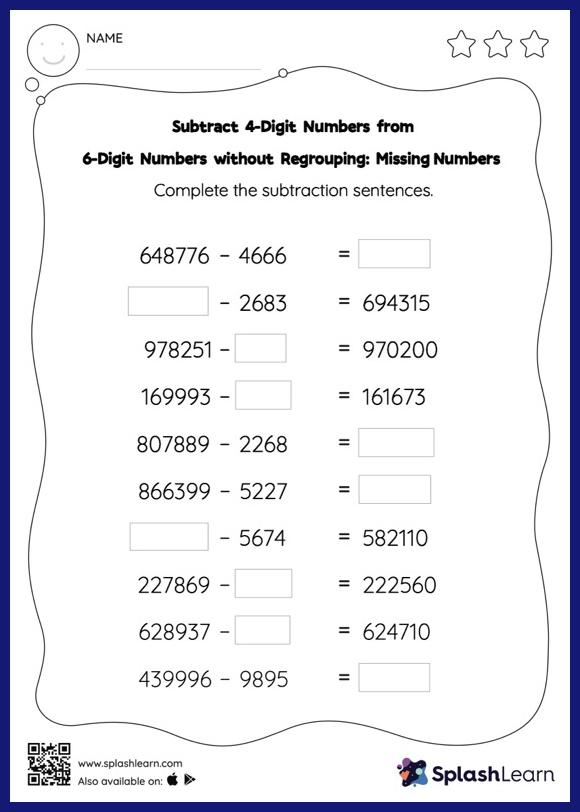# Subtract 4-Digit Numbers from 6-Digit Numbers without Regrouping: Missing Numbers Worksheet

Home > Subtract 4-Digit Numbers from 6-Digit Numbers without Regrouping: Missing NumbersThis worksheet gives students an opportunity to apply the concepts of subtraction to subtract 4-digit numbers from 6-digit numbers without regrouping. Students use the count back strategy or the relationship between addition and subtraction to find the missing number in this worksheet. However, they do not need to regroup numbers to get to the answer in subtract 4-digit numbers from 6-digit numbers without regrouping worksheet. In this worksheet, students practice solving problems written in the horizontal format. How numbers are laid out in a problem affects the method a student employs to solve it. Therefore to develop actual fluency and mastery of multiple strategies, students must practice different formats.